# Diffraction

DiffractionComputer generated intensity pattern formed on a screen by diffraction from a square aperture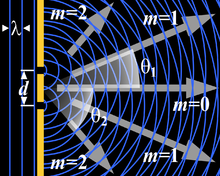Generation of an interference pattern from two-slit diffraction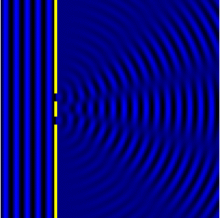Computational Model of an interference pattern from two-slit diffraction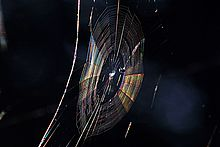Colors seen in a spider web are partially due to diffraction, according to some analyses.

Diffraction refers to various phenomena which occur when a wave encounters an obstacle. Italian scientist Francesco Maria Grimaldi coined the word "diffraction" and was the first to record accurate observations of the phenomenon in 1665. In classical physics, the diffraction phenomenon is described as the apparent bending of waves around small obstacles and the spreading out of waves past small openings. Similar effects occur when light waves travel through a medium with a varying refractive index or a sound wave through one with varying acoustic impedance. Diffraction occurs with all waves, including sound waves, water waves, and electromagnetic waves such as visible light, x-rays and radio waves. As physical objects have wave-like properties (at the atomic level), diffraction also occurs with matter and can be studied according to the principles of quantum mechanics.

Richard Feynman said that

"no-one has ever been able to define the difference between interference and diffraction satisfactorily. It is just a question of usage, and there is no specific, important physical difference between them."

He suggested that when there are only a few sources, say two, we call it interference, as in Young's slits, but with a large number of sources, the process is labelled diffraction.

While diffraction occurs whenever propagating waves encounter such changes, its effects are generally most pronounced for waves where the wavelength is roughly similar to the dimensions of the diffracting objects. If the obstructing object provides multiple, closely spaced openings, a complex pattern of varying intensity can result. This is due to the superposition, or interference, of different parts of a wave that traveled to the observer by different paths (see diffraction grating).

The formalism of diffraction can also describe the way in which waves of finite extent propagate in free space. For example, the expanding profile of a laser beam, the beam shape of a radar antenna and the field of view of an ultrasonic transducer can all be analysed using diffraction equations.

## Examples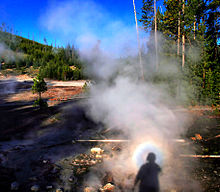Solar glory at the steam from hot springs. A glory is an optical phenomenon produced by light backscattered (a combination of diffraction, reflection and refraction) towards its source by a cloud of uniformly sized water droplets.

The effects of diffraction are often seen in everyday life. The most striking examples of diffraction are those involving light; for example, the closely spaced tracks on a CD or DVD act as a diffraction grating to form the familiar rainbow pattern seen when looking at a disk. This principle can be extended to engineer a grating with a structure such that it will produce any diffraction pattern desired; the hologram on a credit card is an example. Diffraction in the atmosphere by small particles can cause a bright ring to be visible around a bright light source like the sun or the moon. A shadow of a solid object, using light from a compact source, shows small fringes near its edges. The speckle pattern which is observed when laser light falls on an optically rough surface is also a diffraction phenomenon. All these effects are a consequence of the fact that light propagates as a wave.

Diffraction can occur with any kind of wave. Ocean waves diffract around jetties and other obstacles. Sound waves can diffract around objects, which is why one can still hear someone calling even when hiding behind a tree. Diffraction can also be a concern in some technical applications; it sets a fundamental limit to the resolution of a camera, telescope, or microscope.

## History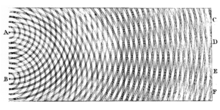Thomas Young's sketch of two-slit diffraction, which he presented to the Royal Society in 1803

The effects of diffraction of light were first carefully observed and characterized by Francesco Maria Grimaldi, who also coined the term diffraction, from the Latin diffringere, 'to break into pieces', referring to light breaking up into different directions. The results of Grimaldi's observations were published posthumously in 1665. Isaac Newton studied these effects and attributed them to inflexion of light rays. James Gregory (1638–1675) observed the diffraction patterns caused by a bird feather, which was effectively the first diffraction grating to be discovered. Thomas Young performed a celebrated experiment in 1803 demonstrating interference from two closely spaced slits. Explaining his results by interference of the waves emanating from the two different slits, he deduced that light must propagate as waves. Augustin-Jean Fresnel did more definitive studies and calculations of diffraction, made public in 1815 and 1818, and thereby gave great support to the wave theory of light that had been advanced by Christiaan Huygens and reinvigorated by Young, against Newton's particle theory.

## MechanismPhotograph of single-slit diffraction in a circular ripple tank

Diffraction arises because of the way in which waves propagate; this is described by the Huygens–Fresnel principle and the principle of superposition of waves. The propagation of a wave can be visualized by considering every point on a wavefront as a point source for a secondary spherical wave. The wave displacement at any subsequent point is the sum of these secondary waves. When waves are added together, their sum is determined by the relative phases as well as the amplitudes of the individual waves so that the summed amplitude of the waves can have any value between zero and the sum of the individual amplitudes. Hence, diffraction patterns usually have a series of maxima and minima.

There are various analytical models which allow the diffracted field to be calculated, including the Kirchhoff-Fresnel diffraction equation which is derived from wave equation, the Fraunhofer diffraction approximation of the Kirchhoff equation which applies to the far field and the Fresnel diffraction approximation which applies to the near field. Most configurations cannot be solved analytically, but can yield numerical solutions through finite element and boundary element methods.

It is possible to obtain a qualitative understanding of many diffraction phenomena by considering how the relative phases of the individual secondary wave sources vary, and in particular, the conditions in which the phase difference equals half a cycle in which case waves will cancel one another out.

The simplest descriptions of diffraction are those in which the situation can be reduced to a two-dimensional problem. For water waves, this is already the case; water waves propagate only on the surface of the water. For light, we can often neglect one direction if the diffracting object extends in that direction over a distance far greater than the wavelength. In the case of light shining through small circular holes we will have to take into account the full three dimensional nature of the problem.

## Diffraction of light

Some examples of diffraction of light are considered below.

### Single-slit diffraction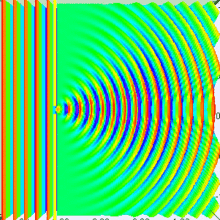Numerical approximation of diffraction pattern from a slit of width equal to wavelength of an incident plane wave in 3D spectrum visualization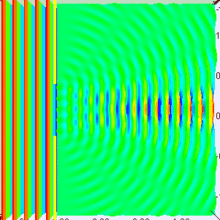Numerical approximation of diffraction pattern from a slit of width equal to five times the wavelength of an incident plane wave in 3D spectrum visualization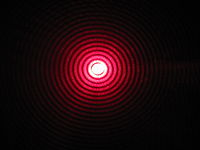Diffraction of red laser beam on the hole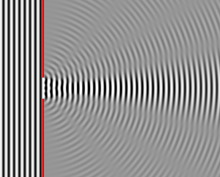Numerical approximation of diffraction pattern from a slit of width four wavelengths with an incident plane wave. The main central beam, nulls, and phase reversals are apparent.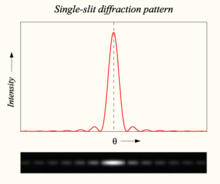Graph and image of single-slit diffraction

A long slit of infinitesimal width which is illuminated by light diffracts the light into a series of circular waves and the wavefront which emerges from the slit is a cylindrical wave of uniform intensity.

A slit which is wider than a wavelength produces interference effects in the space downstream of the slit. These can be explained by assuming that the slit behaves as though it has a large number of point sources spaced evenly across the width of the slit. The analysis of this system is simplified if we consider light of a single wavelength. If the incident light is monochromatic, these sources all have the same phase. Light incident at a given point in the space downstream of the slit is made up of contributions from each of these point sources and if the relative phases of these contributions vary by 2π or more, we may expect to find minima and maxima in the diffracted light. Such phase differences are caused by differences in the path lengths over which contributing rays reach the point from the slit.

We can find the angle at which a first minimum is obtained in the diffracted light by the following reasoning. The light from a source located at the top edge of the slit interferes destructively with a source located at the middle of the slit, when the path difference between them is equal to λ/2. Similarly, the source just below the top of the slit will interfere destructively with the source located just below the middle of the slit at the same angle. We can continue this reasoning along the entire height of the slit to conclude that the condition for destructive interference for the entire slit is the same as the condition for destructive interference between two narrow slits a distance apart that is half the width of the slit. The path difference is given by$\frac{d \sin(\theta)}{2}$ so that the minimum intensity occurs at an angle θmin given by$d\,\sin\theta_\text{min} = \lambda$

where

• d is the width of the slit,
• θmin is the angle of incidence at which the minimum intensity occurs, and
• λ is the wavelength of the light

A similar argument can be used to show that if we imagine the slit to be divided into four, six, eight parts, etc., minima are obtained at angles θn given by$d\,\sin\theta_{n} = n\lambda$

where

• n is an integer other than zero.

There is no such simple argument to enable us to find the maxima of the diffraction pattern. The intensity profile can be calculated using the Fraunhofer diffraction equation as$I(\theta) = I_0 \,\operatorname{sinc}^2 ( d \sin\theta / \lambda )$

where

• I(θ) is the intensity at a given angle,
• I0 is the original intensity, and
• the sinc function is given by sinc(x) = sin(πx)/(πx) if x ≠ 0, and sinc(0) = 1

This analysis applies only to the far field, that is, at a distance much larger than the width of the slit.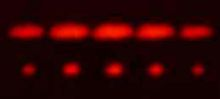2-slit (top) and 5-slit diffraction of red laser light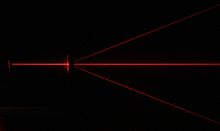Diffraction of a red laser using a diffraction grating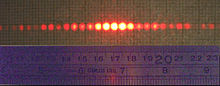A diffraction pattern of a 633 nm laser through a grid of 150 slits

### Diffraction grating

A diffraction grating is an optical component with a regular pattern. The form of the light diffracted by a grating depends on the structure of the elements and the number of elements present, but all gratings have intensity maxima at angles θm which are given by the grating equation$d \left( \sin{\theta_m} + \sin{\theta_i} \right) = m \lambda.$

where

• θi is the angle at which the light is incident,
• d is the separation of grating elements, and
• m is an integer which can be positive or negative.

The light diffracted by a grating is found by summing the light diffracted from each of the elements, and is essentially a convolution of diffraction and interference patterns.

The figure shows the light diffracted by 2-element and 5-element gratings where the grating spacings are the same; it can be seen that the maxima are in the same position, but the detailed structures of the intensities are different.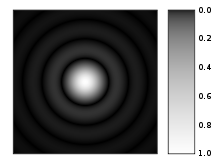A computer-generated image of an Airy disk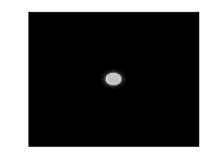Computer generated light diffraction pattern from a circular aperture of diameter 0.5 micrometre at a wavelength of 0.6 micrometre (red-light) at distances of 0.1 cm – 1 cm in steps of 0.1 cm. One can see the image moving from the Fresnel region into the Fraunhofer region where the Airy pattern is seen.

### Circular aperture

The far-field diffraction of a plane wave incident on a circular aperture is often referred to as the Airy Disk. The variation in intensity with angle is given by$I(\theta) = I_0 \left ( \frac{2 J_1(ka \sin \theta)}{ka \sin \theta} \right )^2$

where a is the radius of the circular aperture, k is equal to 2π/λ and J1 is a Bessel function. The smaller the aperture, the larger the spot size at a given distance, and the greater the divergence of the diffracted beams.

### General aperture

The wave that emerges from a point source has amplitude ψ at location r that is given by the solution of the frequency domain wave equation for a point source (The Helmholtz Equation),$\nabla^2 \psi + k^2 \psi = \delta(\bold r)$

where$\delta(\bold r)$ is the 3-dimensional delta function. The delta function has only radial dependence, so the Laplace operator (aka scalar Laplacian) in the spherical coordinate system simplifies to (see del in cylindrical and spherical coordinates)$\nabla ^2\psi= \frac{1}{r} \frac {\partial ^2}{\partial r^2} (r \psi)$

By direct substitution, the solution to this equation can be readily shown to be the scalar Green's function, which in the spherical coordinate system (and using the physics time convention e iωt) is:$\psi(r) = \frac{e^{ikr}}{4 \pi r}$

This solution assumes that the delta function source is located at the origin. If the source is located at an arbitrary source point, denoted by the vector$\bold r'$ and the field point is located at the point$\bold r$, then we may represent the scalar Green's function (for arbitrary source location) as:$\psi(\bold r | \bold r') = \frac{e^{ik | \bold r - \bold r' | }}{4 \pi | \bold r - \bold r' |}$

Therefore, if an electric field, Einc(x,y) is incident on the aperture, the field produced by this aperture distribution is given by the surface integral:$\Psi(r)\propto \int\!\!\!\int_\mathrm{aperture} E_{inc}(x',y')~ \frac{e^{ik | \bold r - \bold r'|}}{4 \pi | \bold r - \bold r' |} \,dx'\, dy',$On the calculation of Fraunhofer region fields

where the source point in the aperture is given by the vector$\bold{r}' = x' \bold{\hat{x}} + y' \bold{\hat{y}}$

In the far field, wherein the parallel rays approximation can be employed, the Green's function,$\psi(\bold r | \bold r') = \frac{e^{ik | \bold r - \bold r' |} }{4 \pi | \bold r - \bold r' |}$

simplifies to$\psi(\bold{r} | \bold{r}') = \frac{e^{ik r}}{4 \pi r} e^{-ik ( \bold{r}' \cdot \bold{\hat{r}})}$

as can be seen in the figure to the right (click to enlarge).

The expression for the far-zone (Fraunhofer region) field becomes$\Psi(r)\propto \frac{e^{ik r}}{4 \pi r} \int\!\!\!\int_\mathrm{aperture} E_{inc}(x',y') e^{-ik ( \bold{r}' \cdot \bold{\hat{r}} ) } \, dx' \,dy',$

Now, since$\bold{r}' = x' \bold{\hat{x}} + y' \bold{\hat{y}}$

and$\bold{\hat{r}} = \sin \theta \cos \phi \bold{\hat{x}} + \sin \theta ~ \sin \phi ~ \bold{\hat{y}} + \cos \theta \bold{\hat{z}}$

the expression for the Fraunhofer region field from a planar aperture now becomes,$\Psi(r)\propto \frac{e^{ik r}}{4 \pi r} \int\!\!\!\int_\mathrm{aperture} E_{inc}(x',y') e^{-ik \sin \theta (\cos \phi x' + \sin \phi y')} \, dx'\, dy'$

Letting,$k_x = k \sin \theta \cos \phi \,\!$

and$k_y = k \sin \theta \sin \phi \,\!$

the Fraunhofer region field of the planar aperture assumes the form of a Fourier transform$\Psi(r)\propto \frac{e^{ik r}}{4 \pi r} \int\!\!\!\int_\mathrm{aperture} E_{inc}(x',y') e^{-i (k_x x' + k_y y') } \,dx'\, dy',$

In the far-field / Fraunhofer region, this becomes the spatial Fourier transform of the aperture distribution. Huygens' principle when applied to an aperture simply says that the far-field diffraction pattern is the spatial Fourier transform of the aperture shape, and this is a direct by-product of using the parallel-rays approximation, which is identical to doing a plane wave decomposition of the aperture plane fields (see Fourier optics).

### Propagation of a laser beam

The way in which the profile of a laser beam changes as it propagates is determined by diffraction. The output mirror of the laser is an aperture, and the subsequent beam shape is determined by that aperture. Hence, the smaller the output beam, the quicker it diverges. Diode lasers have much greater divergence than He–Ne lasers for this reason.

Paradoxically, it is possible to reduce the divergence of a laser beam by first expanding it with one convex lens, and then collimating it with a second convex lens whose focal point is coincident with that of the first lens. The resulting beam has a larger aperture, and hence a lower divergence.

### Diffraction-limited imagingThe Airy disk around each of the stars from the 2.56 m telescope aperture can be seen in this lucky image of the binary star zeta Boötis.

The ability of an imaging system to resolve detail is ultimately limited by diffraction. This is because a plane wave incident on a circular lens or mirror is diffracted as described above. The light is not focused to a point but forms an Airy disk having a central spot in the focal plane with radius to first null of$d = 1.22 \lambda N,\,$

where λ is the wavelength of the light and N is the f-number (focal length divided by diameter) of the imaging optics. In object space, the corresponding angular resolution is$\sin \theta = 1.22 \frac{\lambda}{D},\,$

where D is the diameter of the entrance pupil of the imaging lens (e.g., of a telescope's main mirror).

Two point sources will each produce an Airy pattern – see the photo of a binary star. As the point sources move closer together, the patterns will start to overlap, and ultimately they will merge to form a single pattern, in which case the two point sources cannot be resolved in the image. The Rayleigh criterion specifies that two point sources can be considered to be resolvable if the separation of the two images is at least the radius of the Airy disk, i.e. if the first minimum of one coincides with the maximum of the other.

Thus, the larger the aperture of the lens, and the smaller the wavelength, the finer the resolution of an imaging system. This is why telescopes have very large lenses or mirrors, and why optical microscopes are limited in the detail which they can see.

### Speckle patterns

The speckle pattern which is seen when using a laser pointer is another diffraction phenomenon. It is a result of the superpostion of many waves with different phases, which are produced when a laser beam illuminates a rough surface. They add together to give a resultant wave whose amplitude, and therefore intensity varies randomly.

## Patterns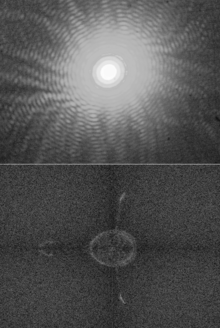The upper half of this image shows a diffraction pattern of He-Ne laser beam on an elliptic aperture. The lower half is its 2D Fourier transform approximately reconstructing the shape of the aperture.

Several qualitative observations can be made of diffraction in general:

• The angular spacing of the features in the diffraction pattern is inversely proportional to the dimensions of the object causing the diffraction. In other words: The smaller the diffracting object, the 'wider' the resulting diffraction pattern, and vice versa. (More precisely, this is true of the sines of the angles.)
• The diffraction angles are invariant under scaling; that is, they depend only on the ratio of the wavelength to the size of the diffracting object.
• When the diffracting object has a periodic structure, for example in a diffraction grating, the features generally become sharper. The third figure, for example, shows a comparison of a double-slit pattern with a pattern formed by five slits, both sets of slits having the same spacing, between the center of one slit and the next.

## Particle diffraction

Quantum theory tells us that every particle exhibits wave properties. In particular, massive particles can interfere and therefore diffract. Diffraction of electrons and neutrons stood as one of the powerful arguments in favor of quantum mechanics. The wavelength associated with a particle is the de Broglie wavelength$\lambda=\frac{h}{p} \,$

where h is Planck's constant and p is the momentum of the particle (mass × velocity for slow-moving particles). For most macroscopic objects, this wavelength is so short that it is not meaningful to assign a wavelength to them. A sodium atom traveling at about 30,000 m/s would have a De Broglie wavelength of about 50 pico meters.

Because the wavelength for even the smallest of macroscopic objects is extremely small, diffraction of matter waves is only visible for small particles, like electrons, neutrons, atoms and small molecules. The short wavelength of these matter waves makes them ideally suited to study the atomic crystal structure of solids and large molecules like proteins.

Relatively larger molecules like buckyballs were also shown to diffract.

## Bragg diffraction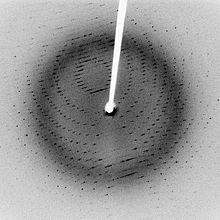Following Bragg's law, each dot (or reflection), in this diffraction pattern forms from the constructive interference of X-rays passing through a crystal. The data can be used to determine the crystal's atomic structure.

Diffraction from a three dimensional periodic structure such as atoms in a crystal is called Bragg diffraction. It is similar to what occurs when waves are scattered from a diffraction grating. Bragg diffraction is a consequence of interference between waves reflecting from different crystal planes. The condition of constructive interference is given by Bragg's law:$m \lambda = 2 d \sin \theta \,$

where

λ is the wavelength,
d is the distance between crystal planes,
θ is the angle of the diffracted wave.
and m is an integer known as the order of the diffracted beam.

Bragg diffraction may be carried out using either light of very short wavelength like x-rays or matter waves like neutrons (and electrons) whose wavelength is on the order of (or much smaller than) the atomic spacing. The pattern produced gives information of the separations of crystallographic planes d, allowing one to deduce the crystal structure. Diffraction contrast, in electron microscopes and x-topography devices in particular, is also a powerful tool for examining individual defects and local strain fields in crystals.

## Coherence

The description of diffraction relies on the interference of waves emanating from the same source taking different paths to the same point on a screen. In this description, the difference in phase between waves that took different paths is only dependent on the effective path length. This does not take into account the fact that waves that arrive at the screen at the same time were emitted by the source at different times. The initial phase with which the source emits waves can change over time in an unpredictable way. This means that waves emitted by the source at times that are too far apart can no longer form a constant interference pattern since the relation between their phases is no longer time independent.

The length over which the phase in a beam of light is correlated, is called the coherence length. In order for interference to occur, the path length difference must be smaller than the coherence length. This is sometimes referred to as spectral coherence, as it is related to the presence of different frequency components in the wave. In the case of light emitted by an atomic transition, the coherence length is related to the lifetime of the excited state from which the atom made its transition.

If waves are emitted from an extended source, this can lead to incoherence in the transversal direction. When looking at a cross section of a beam of light, the length over which the phase is correlated is called the transverse coherence length. In the case of Young's double slit experiment, this would mean that if the transverse coherence length is smaller than the spacing between the two slits, the resulting pattern on a screen would look like two single slit diffraction patterns.

In the case of particles like electrons, neutrons and atoms, the coherence length is related to the spatial extent of the wave function that describes the particle.

Wikimedia Foundation. 2010.

### Look at other dictionaries:

• Diffraction — La diffraction est le comportement des ondes lorsqu elles rencontrent un obstacle qui ne leur est pas complètement transparent ; le phénomène peut être interprété par la diffusion d une onde par les points de l objet. La diffraction se… …   Wikipédia en Français

• diffraction — [ difraksjɔ̃ ] n. f. • 1666; du lat. diffractus, p. p. de diffringere « mettre en morceaux » ♦ Phys. Phénomène optique de déviation des rayons lumineux, au voisinage de corps opaques. ⇒ déflexion, dispersion. Par ext. Phénomène analogue pour d… …   Encyclopédie Universelle

• Diffraction — Dif*frac tion, n. [Cf. F. diffraction.] (Opt.) The deflection and decomposition of light in passing by the edges of opaque bodies or through narrow slits, causing the appearance of parallel bands or fringes of prismatic colors, as by the action… …   The Collaborative International Dictionary of English

• diffraction — 1670s, from Fr. diffraction (17c.) or directly from Mod.L. diffractionem (nom. diffractio), noun of action from pp. stem of L. diffringere break in pieces, from dis apart (see DIS (Cf. dis )) + frangere to break (see FRACTION (Cf. fraction)) …   Etymology dictionary

• Diffraction — des Lichts, s. Beugung des Lichts …   Herders Conversations-Lexikon

• diffraction — DIFFRACTION. sub. fém. Terme d Optique. Inflexion ou détour que subissent les rayons de lumière en rasant la surface d un corps …   Dictionnaire de l'Académie Française 1798

• diffraction — ► NOUN Physics ▪ the process by which a beam of light or other system of waves is spread out as a result of passing through a narrow aperture or across an edge. DERIVATIVES diffract verb diffractive adjective. ORIGIN Latin, from diffringere break …   English terms dictionary

• diffraction — [di frak′shən] n. [ML diffractio < L diffractus: see DIFFRACT] 1. the breaking up of a ray of light into dark and light bands or into the colors of the spectrum, caused by the interference of one part of a beam with another, as when the ray is …   English World dictionary

• Diffraction X — Diffractométrie de rayons X La diffractométrie de rayons X (DRX, on utilise aussi souvent l abréviation anglaise XRD pour X ray diffraction) est une technique d analyse basée sur la diffraction des rayons X sur la matière. La diffraction n ayant… …   Wikipédia en Français

• diffraction — /di frak sheuhn/, n. Physics. 1. the phenomenon exhibited by wave fronts that, passing the edge of an opaque body, are modulated, thereby causing a redistribution of energy within the front: it is detectable in light waves by the presence of a… …   Universalium